Function Repository Resource:

# RatioEqual

Check if two lists are equal as ratios

Contributed by: Wolfram|Alpha Math Team
 ResourceFunction["RatioEqual"][list1,list2,…] returns True if all of the listi are equivalent as ratios.

## Details and Options

Two lists are equivalent as ratios if they have the same length and quotients of corresponding elements all give the same result.
ResourceFunction["RatioEqual"] treats input decimals as exact representations of their rational equivalents. The rational representation of a decimal x is computed as FromDigits[RealDigits[x]].

## Examples

### Basic Examples (2)

Test if two lists are in the same proportion:

 In:=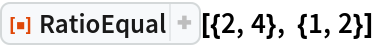Out=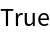Order matters in the comparison of the lists as ratios:

 In:=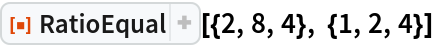Out=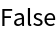### Scope (2)

Test if two ratios involving real and rational numbers are equivalent from a mathematical perspective:

 In:=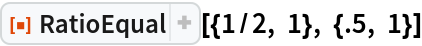Out=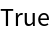Two ratios with symbolic numbers can be equivalent:

 In:=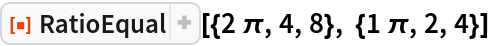Out=## Publisher

Wolfram|Alpha Math Team

## Version History

• 3.0.0 – 23 March 2023
• 2.0.0 – 01 April 2020
• 1.0.0 – 19 March 2020